Basis Functions¶

Data Science School, Nyeri, Kenya¶

15th June 2015 Neil Lawrence¶

We've now seen how we may perform linear regression. Now, we are going to consider how we can perform non-linear regression. However, before we get into the details of how to do that we first need to consider in what ways the regression can be non-linear.

Multivariate linear regression allows us to build models that take many features into account when making our prediction. In this session we are going to introduce basis functions. The term seems complicted, but they are actually based on rather a simple idea. If we are doing a multivariate linear regression, we get extra features that might help us predict our required response varible (or target value), $y$. But what if we only have one input value? We can actually artificially generate more input values with basis functions.

Non-linear in the Inputs¶

When we refer to non-linear regression, we are normally referring to whether the regression is non-linear in the input space, or non-linear in the covariates. The covariates are the observations that move with the target (or response) variable. In our notation we have been using $\mathbf{x}_i$ to represent a vector of the covariates associated with the $i$th observation. The coresponding response variable is $y_i$. If a model is non-linear in the inputs, it means that there is a non-linear function between the inputs and the response variable. Linear functions are functions that only involve multiplication and addition, in other words they can be represented through linear algebra. Linear regression involves assuming that a function takes the form $$f(\mathbf{x}) = \mathbf{w}^\top \mathbf{x}$$ where $\mathbf{w}$ are our regression weights. A very easy way to make the linear regression non-linear is to introduce non-linear functions. When we are introducing non-linear regression these functions are known as basis functions.

Basis Functions¶

Here's the idea, instead of working directly on the original input space, $\mathbf{x}$, we build models in a new space, $\boldsymbol{\phi}(\mathbf{x})$ where $\boldsymbol{\phi}(\cdot)$ is a vector valued function that is defined on the space $\mathbf{x}$.

Remember, that a vector valued function is just a vector that contains functions instead of values. Here's an example for a one dimensional input space, $x$, being projected to a quadratic basis. First we consider each basis function in turn, we can think of the elements of our vector as being indexed so that we have \begin{align*} \phi_1(x) = 1, \\ \phi_2(x) = x, \\ \phi_3(x) = x^2. \end{align*} Now we can consider them together by placing them in a vector, $$\boldsymbol{\phi}(x) = \begin{bmatrix} 1\\ x \\ x^2\end{bmatrix}.$$ This is the idea of the vector valued function, we have simply collected the different functions together in the same vector making them notationally easier to deal with in our mathematics.

When we consider the vector valued function for each data point, then we place all the data into a matrix. The result is a matrix valued function, $$\boldsymbol{\Phi}(\mathbf{x}) = \begin{bmatrix} 1 & x_1 & x_1^2 \\ 1 & x_2 & x_2^2\\ \vdots & \vdots & \vdots \\ 1 & x_n & x_n^2 \end{bmatrix}$$ where we are still in the one dimensional input setting so $\mathbf{x}$ here represents a vector of our inputs with $n$ elements.

Let's try constructing such a matrix for a set of inputs. First of all, we create a function that returns the matrix valued function

In :
import numpy as np # import numpy for the arrays.

"""Take in a vector of input values and return the design matrix associated
with the basis functions."""
return np.hstack([np.ones((n, 1)), x, x**2])

This function takes in an $n\times 1$ dimensional vector and returns an $n\times 3$ dimensional design matrix containing the basis functions. We can plot those basis functions against there input as follows.

In :
# ensure plots appear in the notebook.
%matplotlib inline
import pylab as plt

# first let's generate some inputs
n = 100
x = np.zeros((n, 1))  # create a data set of zeros
x[:, 0] = np.linspace(-1, 1, n) # fill it with values between -1 and 1

fig, ax = plt.subplots(figsize=(12,4))
ax.set_ylim([-1.2, 1.2]) # set y limits to ensure basis functions show.
ax.plot(x[:,0], Phi[:, 0], 'r-', label = '$\phi=1$')
ax.plot(x[:,0], Phi[:, 1], 'g-', label = '$\phi = x$')
ax.plot(x[:,0], Phi[:, 2], 'b-', label = '$\phi = x^2$')
ax.legend(loc='lower right')
Out:
<matplotlib.text.Text at 0x10bc5b510>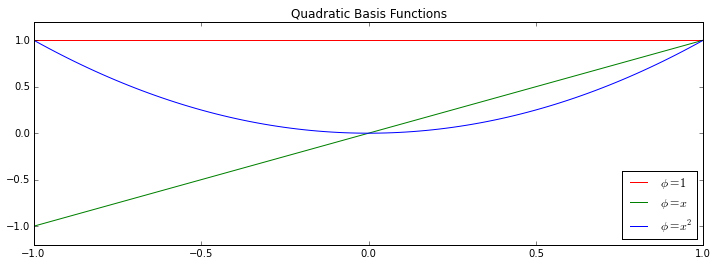The actual function we observe is then made up of a sum of these functions. This is the reason for the name basis. The term basis means 'the underlying support or foundation for an idea, argument, or process', and in this context they form the underlying support for our prediction function. Our prediction function can only be composed of a weighted linear sum of our basis functions.

Different Basis¶

Our choice of basis can be made based on what our beliefs about what is appropriate for the data. For example, the polynomial basis extends the quadratic basis to aribrary degree, so we might define the $j$th basis function associated with the model as $$\phi_j(x_i) = x_i^j$$ which can be implemented as a function in code as follows

In :
def polynomial(x, num_basis=4, data_limits=[-1., 1.]):
Phi = np.zeros((x.shape, num_basis))
for i in xrange(num_basis):
Phi[:, i:i+1] = x**i
return Phi

To aid in understanding how a basis works, we've provided you with a small interactive tool for exploring this polynomial basis. The tool can be summoned with the following command.

In :
import pods
pods.notebook.display_prediction(basis=polynomial, num_basis=4)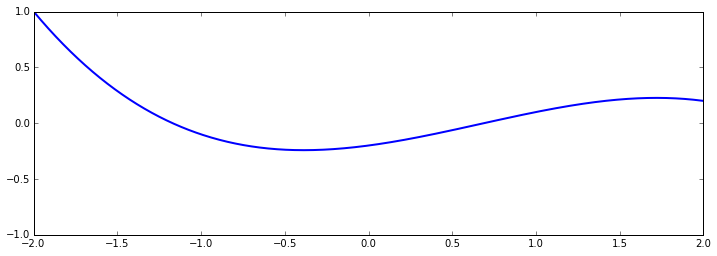In :
fig, ax = plt.subplots(figsize=(12,4))
plt.close(fig)
from IPython.display import display
display(fig)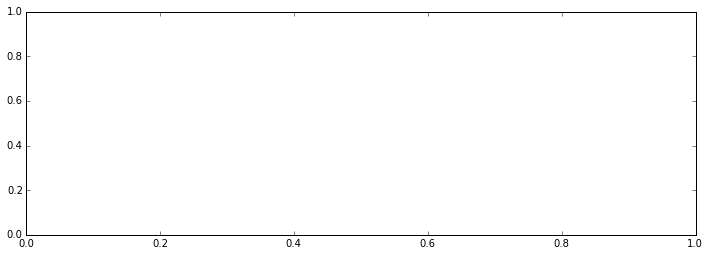Try moving the sliders around to change the weight of each basis function. Click the control box display_basis to show the underlying basis functions (in red). The prediction function is shown in a thick blue line. Warning the sliders aren't presented quite in the correct order. w_0 is associated with the bias, w_1 is the linear term, w_2 the quadratic and here (because we have four basis functions) we have w_3 for the cubic term. So the subscript of the weight parameter is always associated with the corresponding polynomial's degree.

Question¶

Try increasing the number of basis functions (thereby increasing the degree of the resulting polynomial). What do you see as you increase number of basis up to 10? Is it easy to change the function in intiutive ways?

Another type of basis is sometimes known as a 'radial basis' because the effect basis functions are constructed on 'centres' and the effect of each basis function decreases as the radial distance from each centre increases.

In :
def rbf(x, num_basis=4, data_limits=[-1., 1.]):
centres=np.linspace(data_limits, data_limits, num_basis)
width = (centres-centres)/2.
Phi = np.zeros((x.shape, num_basis))
for i in xrange(num_basis):
Phi[:, i:i+1] = np.exp(-0.5*((x-centres[i])/width)**2)
return Phi
In :
pods.notebook.display_prediction(basis=rbf, num_basis=4)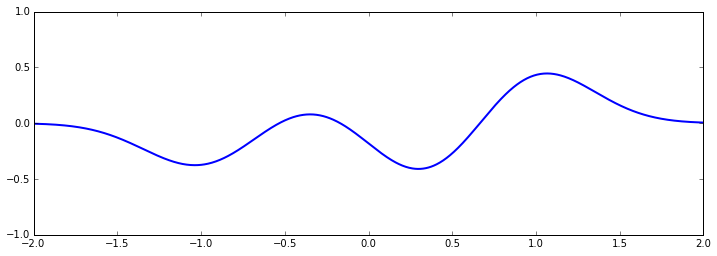Fourier Basis¶

Fourier noticed that any stationary function could be converted to a sum of sines and cosines. A Fourier basis is a linear weighted sum of these functions.

In :
def fourier(x, num_basis=4, data_limits=[-1., 1.]):
tau = 2*np.pi
span = float(data_limits-data_limits)
Phi = np.zeros((x.shape, num_basis))
for i in xrange(num_basis):
count = float((i+1)/2)
frequency = count/span
if i % 2:
Phi[:, i:i+1] = np.sin(tau*frequency*x)
else:
Phi[:, i:i+1] = np.cos(tau*frequency*x)
return Phi

In this code, basis functions with an odd index are sine and basis functions with an even index are cosine. The first basis function (index 0, so cosine) has a frequency of 0 and then frequencies increase to 1, 2, 3, 4 etc every time a sine and cosine are included.

In :
pods.notebook.display_prediction(basis=fourier, num_basis=4)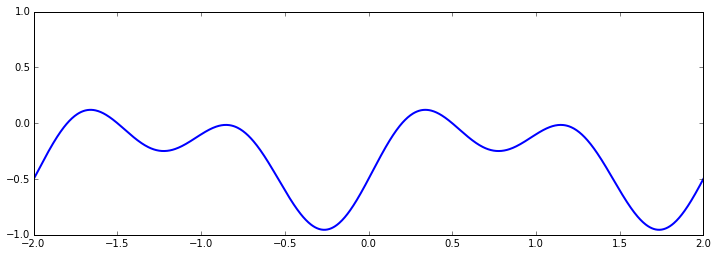Fitting to Data¶

Now we are going to consider how these basis functions can be adjusted to fit the Olympic Marathon data. First we will scale the output of the data to be zero mean and variance 1.

In :
data = pods.datasets.olympic_marathon_men()
y = data['Y']
x = data['X']
y -= y.mean()
y /= y.std()

Redefining the Basis¶

Now we are going to redefine our polynomial basis. Have a careful look at the operations we perform on x to create z. We use z in the polynomial computation. What are we doing to the inputs? Why do you think we are changing x in this manner?

In :
def polynomial(x, num_basis=4, data_limits=[-1., 1.]):
centre = data_limits/2. + data_limits/2.
span = data_limits - data_limits
z = x - centre
z = 2*z/span
Phi = np.zeros((x.shape, num_basis))
for i in xrange(num_basis):
Phi[:, i:i+1] = z**i
return Phi

import pods
#x[:, 0] = np.linspace(1888, 2020, 1000)
fig, ax = plt.subplots(figsize=(12,4))

ax.plot(x, y, 'rx')
pods.notebook.display_prediction(basis=dict(rbf=rbf, polynomial=polynomial, fourier=fourier),
data_limits=(1888, 2020),
fig=fig, ax=ax,
offset=0.,
wlim = (-4., 4., 0.001),
num_basis=4)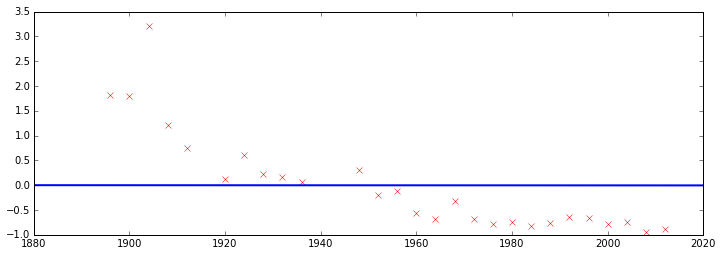Use the tool provided above to try and find the best fit you can to the data. Explore the parameter space and give the weight values you used for the

(a) polynomial basis (b) RBF basis (c) Fourier basis

Which is the easiest to intuitively fit to the te data?

In [ ]:
np.asarray([[1, 2, 3, 4]]).shape

We like to make use of design matrices for our data. Design matrices, as you will recall, involve placing the data points into rows of the matrix and data features into the columns of the matrix. By convention, we are referincing a vector with a bold lower case letter, and a matrix with a bold upper case letter. The design matrix is therefore given by $$\boldsymbol{\Phi} = \begin{bmatrix} 1 & \mathbf{x} & \mathbf{x}^2\end{bmatrix}$$

Non-linear but Linear in the Parameters¶

One rather nice aspect of our model is that whilst it is non-linear in the inputs, it is still linear in the parameters $\mathbf{w}$. This means that our derivations from before continue to operate to allow us to work with this model. In fact, although this is a non-linear regression it is still known as a linear model because it is linear in the parameters, $$f(\mathbf{x}) = \mathbf{w}^\top \boldsymbol{\phi}(\mathbf{x})$$ where the vector $\mathbf{x}$ appears inside the basis functions, making our result, $f(\mathbf{x})$ non-linear in the inputs, but $\mathbf{w}$ appears outside our basis function, making our result linear in the parameters. In practice, our basis function itself may contain its own set of parameters, $$f(\mathbf{x}) = \mathbf{w}^\top \boldsymbol{\phi}(\mathbf{x}; \boldsymbol{\theta}),$$ that we've denoted here as $\boldsymbol{\theta}$. If these parameters appear inside the basis function then our model is non-linear in these parameters.

Question¶

For the following prediction functions see whether the model is linear in the inputs, the parameters or both."""

(a) $f(x) = w_1x_1 + w_2$

(b) $f(x) = w_1\exp(x_1) + w_2x_2 + w_3$

(c) $f(x) = \log(x_1^{w_1}) + w_2x_2^2 + w_3$

(d) $f(x) = \exp(-\sum_i(x_i - w_i)^2)$

(e) $f(x) = \exp(-\mathbf{w}^\top \mathbf{x})$

Fitting the Model Yourself¶

You now have everything you need to fit a non-linear (in the inputs) basis function model to the marathon data.

Choose one of the basis functions you have explored above. Compute the design matrix on the covariates (or input data), x. Use the design matrix and the response variable y to solve the following linear system for the model parameters w. $$\boldsymbol{\Phi}^\top\boldsymbol{\Phi}\mathbf{w} = \boldsymbol{\Phi}^\top \mathbf{y}$$ Compute the corresponding error on the training data. How does it compare to the error you were able to achieve fitting the basis above? Plot the form of your prediction function from the least squares estimate alongside the form of you prediction function you fitted by hand. You can make use of the notebook on regression for assistance in fitting the model.

In [ ]:
#

Lecture on Basis Functions from GPRS Uganda¶

In :
In the last session we showed how rather than computing $\mathbf{X}^\top\mathbf{X}$ as an intermediate step to our solution, we could compute the solution to the regressiond directly through QR-decomposition. With polynomials doing the example with QR decomposition can be critical in obtaining the right answer. Can you rewrite the linear regression using QR decomposition?